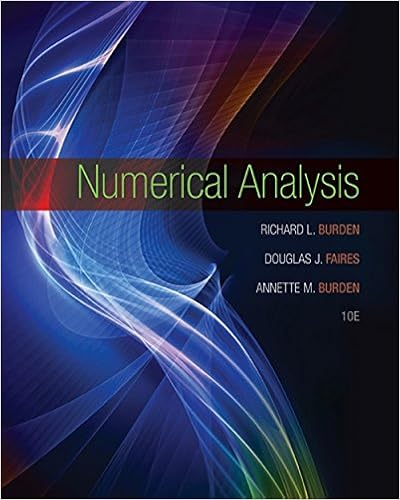# 21C Final Solutions - Calculus Math 21C Fall 2010 Sample...

• Notes
• 16

This preview shows page 1 - 4 out of 16 pages.

##### We have textbook solutions for you!
The document you are viewing contains questions related to this textbook.The document you are viewing contains questions related to this textbook.
Chapter 2 / Exercise 6
Numerical Analysis
Burden/FairesExpert Verified
Calculus : Math 21C, Fall 2010 Sample Final Questions: Solutions 1. Do the following sequences { a n } converge or diverge as n → ∞ ? If a sequence converges, find its limit. Justify your answers. (a) a n = 2 n 2 + 3 n 3 2 n 3 + 3 n 2 ; (b) a n = cos( ); (c) a n = sin( n 2 ) n 2 . Solution.
1
##### We have textbook solutions for you!
The document you are viewing contains questions related to this textbook.The document you are viewing contains questions related to this textbook.
Chapter 2 / Exercise 6
Numerical Analysis
Burden/FairesExpert Verified
2. Do the following series converge or diverge? State clearly which test you use. (a) n =1 n + 4 6 n 17 (b) n =2 n n 4 + 7 (c) n =1 ( 5) n +1 (2 n )! (d) n =3 ln n n (e) 1 1 4 + 1 2 4 1 3 4 + 1 4 4 + 1 5 4 1 6 4 + 1 7 4 1 9 4 + · · · (f) n =1 [ e n e n +1 ]
2
(c) We have c n +1 c n = ( 5) n +2 / (2 n + 2)! ( 5) n +1 / (2 n )! = 5(2 n )! (2 n + 2)! = 5 (2 n + 1)(2 n + 2) . It follows that lim n →∞ c n +1 c n = 0 . Since this limit exists and is less than 1, the series converges absolutely by the ratio test, and therefore it converges. (d) Since ln n > 1 for n 3, we have c n = ln n n > 1 n for n 3 . Since the harmonic series 1 /n diverges, the series diverges by the comparison test. (e) We have | c n | = 1 n 4 so the series converges absolutely, since the p -series with p = 4 con- verges. Therefore the series converges since any absolutely convergent series is convergent. (f) Either note that c n = e n e n +1 = e n (1 e ) diverges to −∞ as n → ∞ , so the series diverges by the n th term test. Or note that the series is a telescoping series of the form c n = b n b n +1 with b n = e n and the limit of b n as n → ∞ does not exist, so the series is a divergent telescoping series. 3
•••